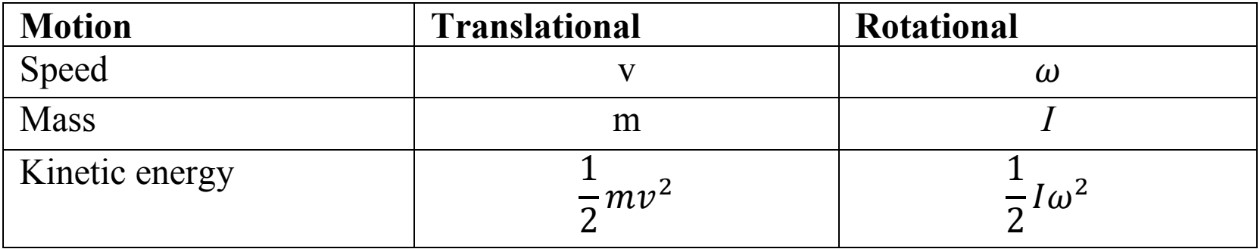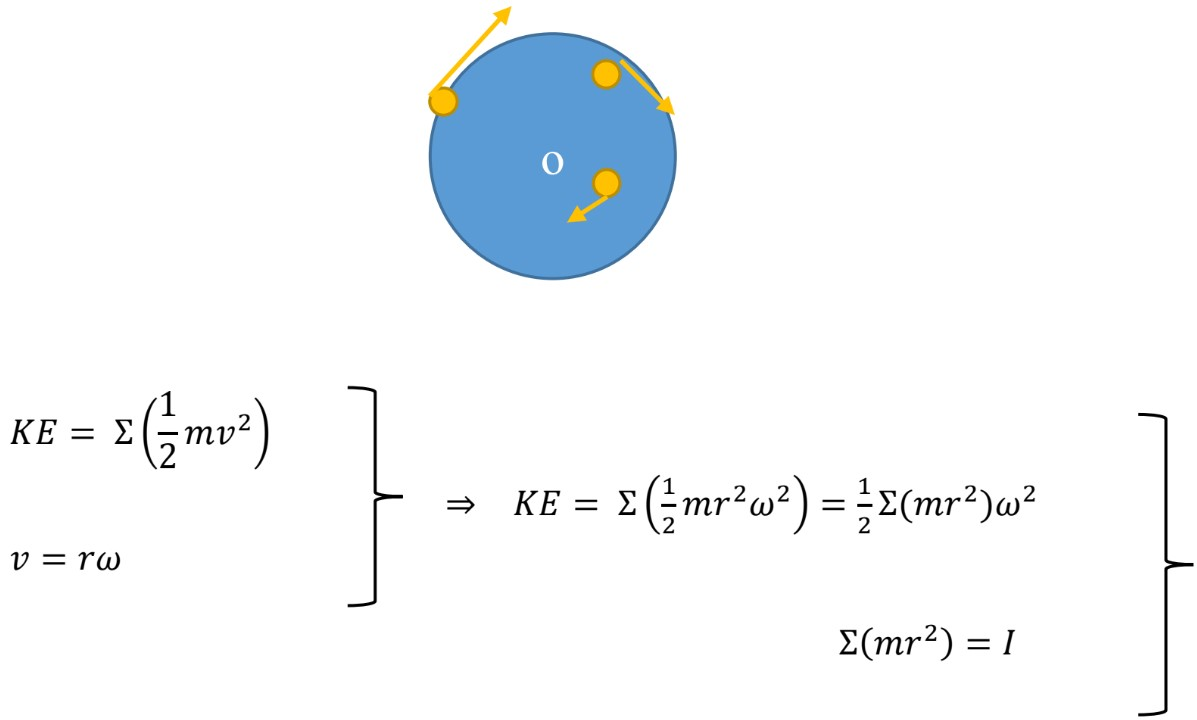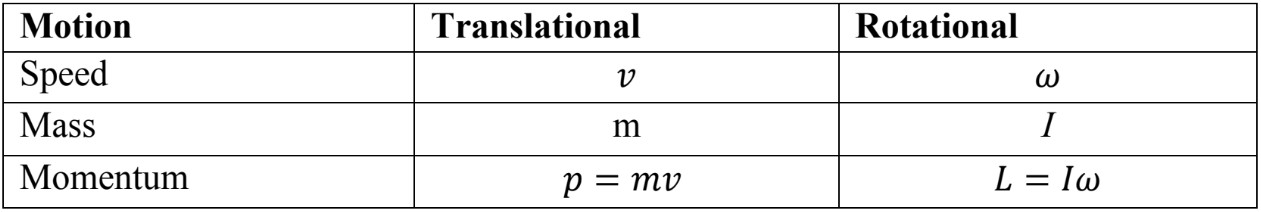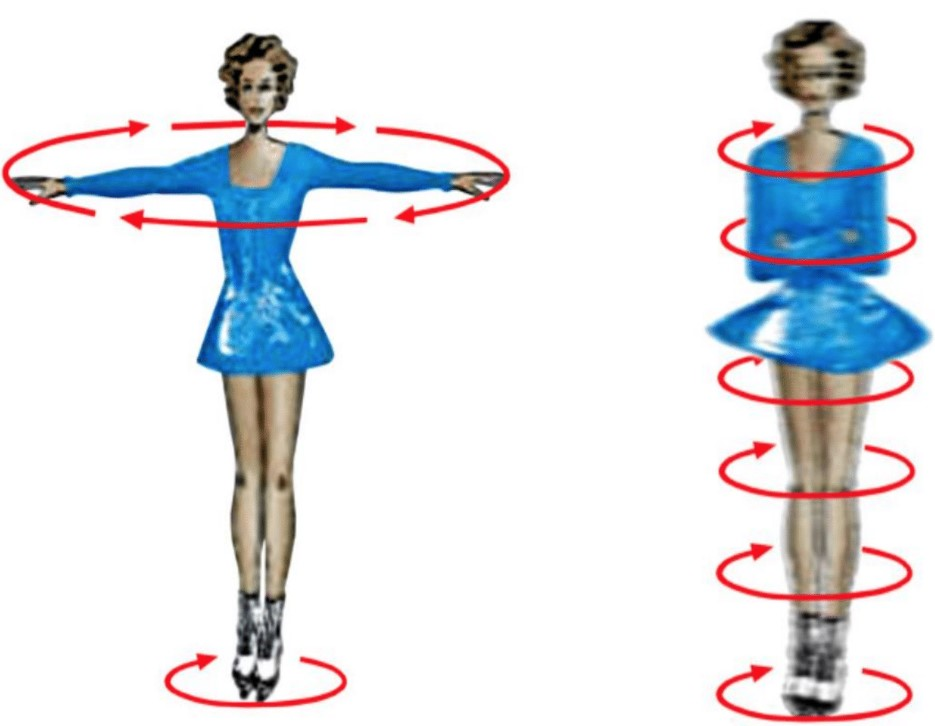# Rotational kinetic energy and angular momentum#### Everything You Need in One Place

Homework problems? Exam preparation? Trying to grasp a concept or just brushing up the basics? Our extensive help & practice library have got you covered.#### Learn and Practice With Ease

Our proven video lessons ease you through problems quickly, and you get tonnes of friendly practice on questions that trip students up on tests and finals.#### Instant and Unlimited Help

Our personalized learning platform enables you to instantly find the exact walkthrough to your specific type of question. Activate unlimited help now!

##### Intros
###### Lessons
1. Rotational kinetic energy
2. Angular momentum
3. Newton's 2nd law for rotation
4. The law of conservation of angular momentum
##### Examples
###### Lessons
1. A bowling ball with a mass on 5.0kg and radius of 12.0cm is rolling down an inclined surface with a negligible friction without slipping. If it starts from rest at a vertical distance of 1.5m, what will be the speed of the ball when it reaches the bottom of the inclined surface?
1. A sphere of radius 24.0 cm and mass of 1.60 kg, starts from the rest and rolls without slipping down a 30.0° incline that is 12.0m long.
1. Calculate the translational and rotational speed when it reaches the bottom.
2. What is the total kinetic energy as it gets to the bottom?
2. Two masses $m_{1}$ = 16.0kg and $m_{2}$ = 24.6kg, are connected by a rope that hangs over a uniform pulley with a mass of 6.50kg and radius of 0.24m. Initially, $m_{1}$ is on the ground and $m_{2}$ rests 2.00m above the ground. If the system is released what will be the speed of $m_{2}$, as it hits the ground?
1. A 2.6kg uniform cylindrical grinding wheel of radius 16cm makes 1600rpm.
1. What is the angular momentum?
2. How much torque is required to stop it in 4.0s?
2. The speed of a skater increases from 2.0 rev every 4.0s to a final rate of 4.0 rev/s. If her moment of inertia was 4.4kg.m2, what is her final moment of inertia?
1. A potter's wheel is rotating around a vertical axis through its center at a frequency of 1.2 rev/s. The wheel can be modeled as a uniform disk of mass 4.0kg and diameter of 0.60m. The potter the throws a 2.1 kg chunk of clay, shaped as a flat disk of radius 6.0cm, onto the center of the rotating wheel. What is the frequency of the wheel after the clay sticks to it?
###### Topic Notes

In this lesson, we will learn:

• How to calculate rotational kinetic energy?
• Definition of angular momentum
• Newton’s 2nd law for rotation
• The law of conservation of angular momentum

Notes:

Rotational Kinetic Energy

• Objects rotating about an axis possess “Rotational Kinetic Energy”
• Objects moving along a straight line possess “Translational Kinetic Energy”.
• Translational kinetic energy is calculated using, $\frac{1}{2}mv^{2}$ , we can convert this formula to rotational kinetic energy question using the rotational motion analogues:

•If we consider a rotating ball, every point on the ball is rotating with some speed.
The ball is made up many tiny particles, each of mass “$m$”. let’s take “$r$” to be the distance of any one particle from the axis of rotation (O);$\Rightarrow \,$ rotational $KE = \frac{1}{2}I\, \omega^{2}$

• The center of mass of a rotating object might undergo translational motion (a sphere rolling down an incline), in this case we have to consider both rotational and translational kinetic energy.

• $KE = \frac{1}{2}M v^{2} \, + \, \frac{1}{2}I_{CM \;} \omega^{2}$

$M$: total mass of the object
$I_{CM}$: moment of inertia about the axis through center of mass
$v$: translational speed
$\omega$: angular speed

Angular Momentum
In the previous section, we used the rotational analogue to translate the translational kinetic energy to rotational kinetic energy.
In like manner, the linear momentum can be changed to angular momentum, using the rotational analogues;$L$: is the angular momentum with a standard unit of kg.m2/s

Newton’s 2nd Law for Rotation

The Newton’s 2nd law also can be written in terms of rotational analogues;

$\sum F = \, ma \, =$ $\large \frac{m \Delta v}{\Delta t}$ $=$ $\large \frac{\Delta p}{\Delta t} \quad$, in translational motion “Force” causes linear acceleration

$\qquad \quad$ Similarly for rotational motion , $\sum =$ $\large \frac{\Delta L }{\Delta t}$

$\sum \tau = \,$ $\large \frac{\Delta L}{\Delta t}$ $=$ $\large \frac{I \Delta \omega}{\Delta t}$ $\, = I \propto \quad$, in rotational motion “Torque” causes rotational acceleration

$\sum F = ma \quad$ Newton’s 2nd Law in Translational Motion
$\sum \tau = I \propto \quad$ Newton’s 2nd Law in Rotational Motion

The law of Conservation of Angular Momentum

The law of conservation of angular moment states that;

“The total angular momentum of a rotating object remains constant if the net torque acting on it is zero.”

$\sum \tau = 0 \; \Rightarrow \; L_{i} = L_{f} \; \Rightarrow \; I_{i \omega i} = I_{f \omega f}$

Example: A skater doing a spin on ice, illustrating conservation of angular momentum.Open arms: $\qquad L_{i} = I \omega_{i} = mr^{2}_{i} \omega_{i}$
Closed arms:   $\qquad L_{f} = I \omega_{f} = mr^{2}_{f} \omega_{f}$

When the arms of the skater are tucked in, the mass is not changing, but the radius of rotation decreases; $r_{f}$ < $r_{i}$, therefore;  $mr^{2}_{f}$ < $mr^{2}_{i}$

According to the law of conservation of angular momentum; $L_{i} = L_{f}$

$L_{i} = L_{f}$

$\qquad \Rightarrow \quad \omega_{f}$ > $\omega_{i} \;$ “the result would be higher angular velocity”

$mr^{2}_{f}$ < $mr^{2}_{i}$